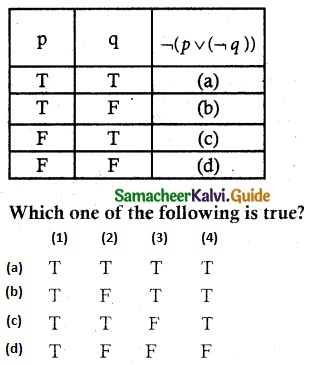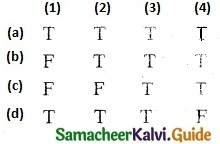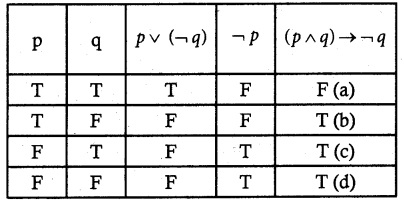Tamilnadu State Board New Syllabus Samacheer Kalvi 12th Maths Guide Pdf Chapter 12 Discrete Mathematics Ex 12.3 Text Book Back Questions and Answers, Notes.

## Tamilnadu Samacheer Kalvi 12th Maths Solutions Chapter 12 Discrete Mathematics Ex 12.3

Choose the most suitable answer from the given four alternatives:

Question 1.
A binary operation on a set Sis a function from
(a) S → S
(b) (S × S) → S
(c) S → (S × S)
(d) (S × S) → (S × S)
Solution:
(b) (S × S) → S

Question 2.
Subtraction is not a binary operation in
(a) R
(b) Z
(c) N
(d) Q
Solution:
(c) N
Hint:
Let 1, 2 ∈ N
1 * 2 = 1 – 2 = -1 ∉ NQuestion 3.
Which one of the following is a binary operation on N?
(a) Subtraction
(b) Multiplication
(c) Division
(d) All the above
Solution:
(b) Multiplication

Question 4.
In the set R of real numbers ‘*’ is defined as follows. Which one of the following is not a binary operation on R ?
(a) a * b = min (a.b)
(b) a * b = max (a, b)
(c) a * b = a
(d) a * b = ab
Solution:
(d) a * b = ab
Hint:
Since -2, 1/2 ∈ R , but (-2)1/2 ∉ R.Question 5.
The operation * defined by a * b = $$\frac { ab }{ 7 }$$ is not a binary operation on
(a) Q+
(b) Z
(c) R
(d) C
Solution:
(b) Z
Hint:
a * b = $$\frac { ab }{ 7 }$$ ∉ Z
as Z is set of all integers.

Question 6.
In the set Q define a $$\bigodot$$ b = a + b + ab. For what value of y, 3 $$\bigodot$$ (y $$\bigodot$$ 5) = 7?
(a) y = $$\frac { 2 }{ 3 }$$
(b) y = $$\frac { -2 }{ 3 }$$
(c) y = $$\frac { -3 }{ 2 }$$
(d) y = 4
Solution:
(b) y = $$\frac { -2 }{ 3 }$$
Hint:
a $$\bigodot$$ b = a + b + ab
Given 3 $$\bigodot$$ (y $$\bigodot$$ 5) = 7
3 $$\bigodot$$ (y + 5 + 5y) = 7
3 $$\bigodot$$ (6y + 5) = 7
3 + 6y + 5 + 3 (6y + 5) = 7
8 + 6y + 18y + 15 = 7
24 y = 7 – 23 = -16
y = $$\frac { -16 }{ 24 }$$ = $$\frac { -2 }{ 3 }$$Question 7.
If a * b = $$\sqrt { a^2+b^2 }$$ on the real numbers then * is
(a) Commutative but not associative
(b) Associative but not commutative
(c) Both commutative and associative
(d) Neither commutative nor associative
Solution:
(c) Both commutative and associative
Hint:∴ (a * b) * c = a * (b * c)
* is associative

Question 8.
Which one of the following statements has the truth value T?
(a) sin x is an even function.
(b) Every square matrix is non-singular
(c) The product of complex number and its conjugate is purely imaginary
(d) $$\sqrt{5}$$ is an irrational number
Solution:
(d) $$\sqrt{5}$$ is an irrational numberQuestion 9.
Which one of the following statements has truth value F?
(a) Chennai is in India or √2 is an integer.
(b) Chennai is in India or √2 is an irrational number.
(c) Chennai is in China or √2 is an integer.
(d) Chennai is in China or √2 is an irrational number.
Solution:
(c) Chennai is in China or √2 is an integer.

Question 10.
If a compound statement involves 3 simple statements, then the number of rows in the truth table is ……….
(a) 9
(b) 8
(c) 6
(d) 3
Solution:
(b) 8
Hint:
(i.e.) 23 = 8Question 11.
Which one is the inverse of the statement (p v q) → (p ∧ q)?
(a) (p ∧ q) → (p v q)
(b) ¬(p v q) → (p ∧ q)
(c) (¬P v ¬q) → (¬p ∧ ¬q)
(d) (¬p ∧ ¬q) → (¬p v ¬q)
Solution:
(d) (¬p ∧ ¬q) → (¬p v ¬q)
Hint:
(p v q) → (p ∧ q)
¬(p v q) → ¬(p ∧ q)
(¬P ∧ ¬q) → (¬p v ¬q)

Question 12.
Which one is the contrapositive of the statement (p v q) → r?
(a) ¬r → (¬p ∧ ¬q)
(b) ¬r → (p v q)
(c) r → (p ∧ q)
(d) p → (q v r)
Solution:
(a) ¬r → (¬p ∧ ¬q)
Hint:
(p v q) → r
Contrapositive is ¬r → ¬(p v q)
¬r → (¬p ∧ ¬q)Question 13.
The truth table for (p ∧ q) v ¬q is given belowSolution:
(c) (1) T, (2) T, (3) F, (4) T
Hint:1 = T, 2 = T, 3 = F, 4 = T

Question 14.
In the last column of the truth table for ¬(p v ¬q) the number of final outcomes of the truth value ‘F’ is
(a) 1
(b) 2
(c) 3
(d) 4
Solution:
(c) 3
Hint:Number of ‘F’ in last column is 3Question 15.
Which one of the following is incorrect? For any two propositions p and q, we have
(a) ¬(p v q) ≡ ¬p ∧ ¬q
(b) ¬(p ∧ q) ≡ ¬p v ¬q
(c) ¬(p v q) ≡ ¬p v ¬q
(d) ¬(¬p) ≡ p
Solution:
(c) ¬(p v q) ≡ ¬p v ¬q

Question 16.Which one of the following is correct for the truth value of (p ∧ q) → ¬pSolution:
(b) (1) F, (2) T, (3) T, (4) T
Hint:Question 17.
The dual of ¬(p v q) v [p v(p ∧ ¬r)] is
(a) ¬(p ∧ q) ∧ [p v(p ∧ ¬r)]
(b) (p ∧ q) ∧ [p v(p v ¬r)]
(c) ¬(p ∧ q) ∧ [p ∧ (p ∧ r)]
(d) ¬(p ∧ q) ∧ [p ∧ (p v ¬r)]
Solution:
(d) ¬(p ∧ q) ∧ [p ∧ (p v ¬r)]
Hint:
Dual is obtained by changing ∧ into v and vice versa.

Question 18.
The proposition $$p \wedge(\neg p \vee q)]$$ is ……..
(a) a tautology
(c) logically equivalent to $$p \wedge q$$
(d) logically equivalent to $$p \vee q$$
Solution:
(c) logically equivalent to $$p \wedge q$$Question 19.
Determine the truth value of each of the following statements:
(a) 4 + 2 = 5 and 6 + 3 = 9
(b) 3 + 2 = 5 and 6 + 1 = 7
(c) 4 + 5 = 9 and 1 + 2 = 4
(d) 3 + 2 = 5 and 4 + 7 = 11Solution:
(a) (1) F, (2) T, (3) F, (4) T
Hint:
(1) F and T = F
(2) T and T =T
(3) T and F = F
(4) T and T = T

Question 20.
Which one of the following is not true?
(a) Negation of a statement is the statement itself.
(b) If the last column of the truth table contains only T then it is a tautology.
(c) If the last column of its truth table contains only F then it is a contradiction
(d) If p and q are any two statements then p ⟷ q is a tautology.
Solution:
(d) If p and q are any two statements then p ⟷ q is a tautology.